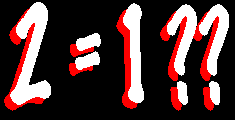Proving 2 Equals 1I ONCE used to be very interested in mathematics, but found myself completely disillusioned to a point where I now realise that virtually all of the advanced mathematics I studied at senior high school and university is totally useless to me. Let me explain via an example. All through high school I was told that it is not possible to find the square root of a negative number because any number - be it positive or negative - will always produce a positive answer when squared. But then came "complex numbers". They actually said that the square root of minus one (-1) was i. Why? Because the professor said so. Everybody knew it was rubbish, but we had to learn it anyway. Perhaps there are a few mathematicians out there who would like to defend their one love and disprove the following. Can it be possible that 2 equals 1? Using simple algebra and illusion, I can show that this is the case. Firstly, let's say that: x = 1 Therefore: x² = x x² - 1 = x -1 Factorising: (x - 1)(x + 1) = x - 1 Dividing through: x + 1 = 1 Substituting: 2 = 1 How is this so?Enigma :

Here's my solution and/or comments :